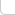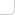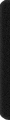# 某島

… : "…アッカリ～ン . .. . " .. .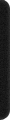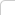July 25, 2015

## HDU 5306. Gorgeous Sequence

### Breif description:

There is a sequence a of length n. We use ai to denote the i-th element in this sequence. You should do the following three types of operations to this sequence.

• 0 x y t: For every x≤i≤y, we use min(ai,t) to replace the original ai’s value.（區間取 min）
• 1 x y: Print the maximum value of ai that x≤i≤y.（區間求最大値）
• 2 x y: Print the sum of ai that x≤i≤y.（區間求和）

### Analysis:

• “>http://codeforces.com/contest/444/problem/C

• 暴力的線段樹

...
void upd(int x, int l, int r){  //上傳信息
T[x].ss = T[lx].ss + T[rx].ss;
T[x].mx = max(T[lx].mx, T[rx].mx);
}

void pnc(int x, int l, int r, int t){ //打標記
if (T[x].mx <= t) return;

T[x].tag = t;

if (l < r){
pnc(lx, l, ml, t); pnc(rx, mr, r, t);
upd(xx);
}
else{
T[x].ss = T[x].mx = t;
}
}

void rls(int x, int l, int r){ //下放標記，因為我們是暴力打標記的。。所以暫時不需要。。
//pnc(lc, T[x].tag); pnc(rc, T[x].tag);
}


我們發現主要瓶頸是打標記這個步驟，因為在標記作用上的時刻，我們不知道它的子樹中，有多少節點將會被這個標記影響。
因此需要遞歸到子樹中去。。。

注意到這題中我們的 tag 標記是單調的，只需要留意子樹中更小的標記。

   4            4
2   6   ->   2   -
1 3 5 7      1 -


雖然這樣第一行加了剪枝，然而並沒有什麼卵用。最壞情況下，遞減的修改根節點，每次我們需要遞歸整棵樹，複雜度 O(n)，和直接用數組暴力的複雜度一樣。。。
。。。回憶 http://codeforces.com/contest/444/problem/C 。。。

我們注意到，在這種情況下，所有節點的 tag 信息總是相同的，我們理應利用這個信息，不要每次遞歸到整個子樹中去。
而這要求我們多維護一個額外信息。。。

struct rec{
LL ss; int mx, cv;
int tag;
} T[N];


這裡 cv 表示，這個區間中有多少個結點，已被子樹中的 tag 影響。

我們有：

void upd(int x, int l, int r){
T[x].ss = T[lx].ss + T[rx].ss;
T[x].mx = max(T[lx].mx, T[rx].mx);
T[x].cv = T[lx].cv + T[rx].cv;
}

void pnc(int x, int l, int r, int t){
if (T[x].tag != Dummy && T[x].tag <= t) return;

T[x].tag = t;
if (T[x].cv != r-l+1){
T[x].mx = t;
T[x].ss += (LL) t * ((r-l+1)-T[x].cv);
T[x].cv = r-l+1;
}
}

void rls(int x, int l, int r){
if (T[x].tag != Dummy){
pnc(lc, T[x].tag); pnc(rc, T[x].tag);
}
}



。。。這樣還是我們熟悉的普通線段樹的寫法。。。複雜度是對的。。但是問題在於，在打標記的時候，我們其實並不能直接維護 cv
因此在每次修改的時候，我們還需要添加一個 fix 函數，清理子樹中所有大於等於 t 的標記。

void Insert(int x, int l, int r){

if (T[x].mx <= t) return;

if (b < l || r < a) return;
if (a <= l && r <= b){
fix(xx, t);
if (l == r){
T[x].ss = T[x].mx = T[x].tag = t;
T[x].cv = 1;
}
else{
upd(xx);
}
pnc(xx, t);
}
else{
rls(xx);
Insert(lc); Insert(rc);
upd(xx);
}
}


。。。這樣我們還剩 fix 函數需要考察。。。

void fix(int x, int l, int r, int t){

if (T[x].mx < t) return;

if (T[x].tag >= t){
T[x].tag = Dummy;
}
if (l == r){
T[x].ss = T[x].mx = T[x].tag = T[x].tag;
T[x].cv = T[x].tag != Dummy;
}
else{
rls(xx);
fix(lc, t); fix(rc, t);
upd(xx);
}
}


。。。此時它已經和我們最初的方法有了很大的不同。。。。。

初看起來，每次 Insert 操作我們可能調用 O(log(n))fix 函數，每次 fix 的複雜度又可能高達 O(n)

我們對其進行均攤分析！

當一個標記被清理成 Dummy 時，其子樹中所有標記也都被賦成 Dummy，並且它的 mx = 0
我們考察最上面的那些 Dummy 標記，稱之為 封閉節點
我們設置 封閉節點mx 値等於 0，因而它們不會參與到 fix 函數的遞歸中去。

• 對於每次 Insert 操作，最多可能形成 O(n)封閉節點
• 對於每次 Insert 操作，最多只會打開 O(logn)封閉節點

因此總的時間複雜度仍為 O(nlogn)-> 完整代碼